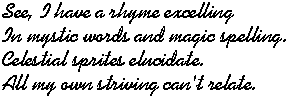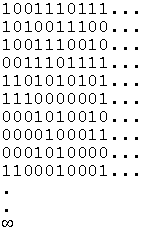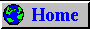The World of NumbersThis page, like the companion Science page, contains items and links that appeal to me for one reason or another. Here you will find various topics related to mathematics and statistics, including links to reference material, tutorials, Macintosh freeware, and miscellaneous tidbits as well as to other sites like this one. The list is not meant to be comprehensive and the selection is based, as always, on my personal perspective. Thus, this page will always be under construction!

Mathematics

It would be difficult to find any other subject, of comparable relevance and importance, about which so many are so proud of their ignorance. Even the most fastidious individuals, who would never tolerate the unforgivable solecism of a split infinitive, will not hesitate to proclaim, to one and all, their utter inadequacy when it comes to anything pertaining to numbers. This includes activities even as mundane as rescaling a recipe, reading a weather map, or computing the total cost of a mortgage. Tell people that the Sun is far away and they will nod their head and try to look intelligent. Say that it is 93,000,000 miles away and their eyes will start to glaze over as they search for some means of escape.

If you are reading this page, then you are likely in that happy minority who know better. Therefore, you might find things here that you will appreciate.

A Real Rogue's Gallery

Sometimes, just looking at numbers in different ways can be both interesting and insightful. See what you think of these:
• What is this number?
Here is the ultimate bottoms-up approach to analysis. You have computed a number to, say, 15 decimal places but you have no idea at all what it might look like symbolically. Click this link and find out. I have saved a lot of work using this excellent reference.
• All about Pi
Everything you ever wanted to know about Pi and then some. Check out the Java computation!
• The Golden Section
Included here is information about the Golden Section (or Ratio, or Mean) as well as Fibonacci numbers, one of the origins of one of my favorite numbers.
• Feigenbaum's Constant
Actually, there are more than one of these but who's counting?
• Lots More...

Let's Not Forget the Integers

lest they feel neglected.
• Mysterious sequences
Have you ever wondered if a sequence of integers followed some simple rule? Well, look it up.
• Pascal's Triangle
You would be amazed at how often this pattern shows up in so many different places for so many different reasons.
• Prime numbers
Look at a list or jump to this prime site.
• Perfect numbers
According to a mathematician's concept of perfection.

Famous Theorems, etc.

Theorems are more than just something you have to memorize in geometry class. They represent Truth in a way that is otherwise absent from life in general. A theorem, once proven, remains 100% true forever. Occasionally, a theorem will gain almost universal recognition due either to its elegance and beauty or its utility. Here are some of my favorites:
• Pythagorean Theorem
Easily the most famous of all mathematical theorems—the one that nearly everyone knows. And, it seems, the most popular candidate for new proofs. Here is a page showing lots of interesting proofs.

The prettiest illustration (not proof) of this theorem that I have ever seen was in the Ontario Science Center in Toronto, Canada. The exhibit showed a large right triangle, made out of transparent plastic, about an inch thick. Each side of the triangle was also one side of a square. Unlike the triangle, these three squares were hollow and connected together. The square on the hypotenuse was filled with water colored blue and the whole figure rotated 180 degrees about the hypotenuse every ten seconds. You can guess the rest.

• The Infinity of Primes
Euclid showed, around 300 BC, that there are an infinity of prime numbers, that is, positive integers that cannot be divided by any other integer greater than one and less than the prime itself (without having a remainder left over).

This demonstration is both elegant and straightforward. Just start with any prime number, M, then construct the number
N = M! + 1 = [M*(M-1)*(M-2)*(M-3)*...*2*1] + 1.
So, what can one say about N? Obviously, N is bigger than M. It is also obvious that N cannot have any factor, from 2 through M, because there will always be a remainder of one, by construction. But, is N prime?

Answer: Either N is, itself, a prime number or it has a prime factor, J, which must necessarily be bigger than M (see last paragraph). Either way, we have found a new prime number, N or J, that is bigger than M. Since we could repeat this process indefinitely, with each new prime that we find, there must be an infinity of primes.

This proof is an illustration of one form of mathematical induction.

• Fermat's Last Theorem
This is probably the second most famous mathematical theorem. It is an integer generalization of the Pythagorean Theorem, as described here. It has finally been proven!

Footnote:
Several years ago, at a party, another guest started telling me about his "proof" of Fermat's Last Theorem. He said that he had tried a lot of integers and, so far, the theorem seemed to be correct. I asked how long he intended to keep this up. I do not recall his answer verbatim but got the impression that, when his patience was exhausted, that would constitute some kind of proof. Naturally, my pedagogical instincts took over and I tried to explain that, in mathematics, this was not quite how it was done, that the theorem was supposed to be perfectly general so the proof must be likewise.

He didn't get it. Most people don't.
I should have shown him something like the following proof that numbers can sometimes be irrational.

• Existence of Irrational Numbers
As the name suggests, an irrational number is a number that can never be expressed, exactly, as a ratio of integers, no matter which two integers you pick. As with the Pythagorean Theorem, proving this is something of a cottage industry. Everyone seems to have his/her favorite proof. I imagine that a thousand people probably "discovered" this before me but, FWIW, here is my proof that the common logarithm of 5 (= 0.69897...) must be irrational:What? You say that the Q.E.D. (quod erat demonstrandum) is not obvious? Are you sure??
In that case, here is the explanation.

Incidentally, this is an excellent counterexample to the proposition that you cannot prove a negative. This proof shows conclusively that the given hypothesis is impossible.

• Fundamental Theorem of Algebra
As succinctly stated on this page, the FTA asserts that
Every polynomial of degree N, with complex coefficients, has exactly N roots.
Note that the roots need not be distinct! Since the complex numbers contain the real numbers, it follows that a polynomial of degree N, with real coefficients, also has N roots.

Thus, for example, the number 8 has three cube roots, all solutions of x3 = 8. How many can you find?

Finally, if a polynomial is written with the exponents in numerical order and the coefficients are all real numbers, then Descartes' Rule of Signs states that there are at most M positive, real roots where M is the number of sign changes in the sequence of coefficients.

• Cantor's Diagonal Proof
My all-time favorite!

This is a proof that, even though there are an infinity of positive integers, these are still not enough to count the irrational numbers. In other words, the infinity of irrationals is "larger" than the infinity of positive integers. This, of course, means that there are "levels" of infinity. A truly mind-boggling concept!

The proof is actually quite simple (in hindsight) and may be paraphrased as follows:
First, assume that the fractional parts of all the irrational numbers are listed in a table, in no particular order. Also, to keep things simple, let these numbers be written in binary notation, as shown below.Realize that, since these numbers are irrational, their fractional parts neither terminate nor repeat. [If they did either, then they would be rational.] Thus, there are an infinity of columns in the array above. Likewise, the rows could be numbered and, since there are an infinity of positive integers to count with, you might think that this would be enough. The big question, then, is whether or not this infinity of rows contains all of the irrational numbers or if, perhaps, one or more of the latter is missing.

Now for the clever part.
Construct an irrational number, IRR, by going through the list above and flipping all of the diagonal elements. In other words, change digit from 1 to 0, digit from 0 to 1, etc., and imagine going through the entire diagonal in this fashion. Is the resulting irrational number, IRR, anywhere in the list (which is infinite and, therefore, presumably complete)?

The answer is clearly No. IRR cannot be the first number listed because their first digits are different. It cannot be the second number listed because their second digits are different. In general, IRR cannot be the kth number in the list because the two will differ, at least, in digit k. Even though there are an infinity of irrational numbers in our list, nevertheless, IRR is missing! In plain English, there are "more" irrationals than there are positive integers.

Further commentary would be superfluous. You will just have to think about it, or go here for additional information.

• Gödel's Proof
This is a proof that any set of axioms sufficient to describe arithmetic must yield unprovable statements. An obvious corollary is that arithmetic can never be shown to be self-consistent. Naturally, there are implications for sets of axioms in other fields besides mathematics. I will not attempt to give a simple rendition. If you would like one, try this page.
• Four-color Theorem
A pleasant alternative to this otherwise black-and-white synopsis. Briefly, this proof shows that, no matter what sort of strangely-shaped countries you might imagine (on a flat surface), four colors are always sufficient to paint the map without having any two adjacent countries of the same color. Check it out!
• Goldbach's Conjecture
This is not "officially" a theorem, more of a speculation. Simply put, it states that every positive, even integer is the sum of exactly two prime numbers. For example, 36 = 17 + 19; 64 = 59 + 5; 100 = 97 + 3; etc. [Sometimes, the even number two is excluded because the number one is not always considered prime.]

This conjecture has never been proven or disproven and, if you can find a counterexample, then you will become famous! Here is a link to further information.

Tutorials

Here are some leading references to math tutorials and exercises on the 'Net. Obviously, you can find a lot more using almost any search engine. These sites reference many further links and are provided just to get you started.
• Felecia's Algebra Tutorial
is a good place to start on those wonderful word problems and graphs.
• Webtrig
introduces you to trigonometry. It is in PDF and requires the (free) Adobe Acrobat plugin.
• e-Calculus
is a PDF tutorial for Calculus I.
• S.O.S. Mathematics
covers a lot of ground, from basic algebra to complex variables and matrix algebra.

Macintosh Freeware

These are just a few of the many free software packages readily available for the numerate Mac user.
• Math Masters
is an online game for kids to improve their arithmetic skills.
• More Calculators...
... an embarrassment of riches at the InfoMac site.
• NumberCrunch
evaluates algebraic expressions that you type into a parser.
is, according to the developer, "a general purpose graphing scientific calculator. It uses a text window rather than simulating buttons on a hand held calculator. This live scratchpad interface allows you to see and edit your entire calculation." The latter is an understatement. MathPad is really a very simplified version of Mathematica or MatLab. The package includes enough examples to give you the general idea.

The following are sites of general interest:
• Mathworld
probably the best and often cited.
• Ask Dr. Math
is a K-12 resource.
• Math2.org
All the formulas you could ever want, and pictures, too!
• The Integrator
Type in a formula and Mathematica® will integrate it for you, if possible.

Statistics

Statistics, albeit a discipline in its own right, is primarily an ever-improving cumulation of mathematical tools for extracting information from data. To a physical scientist, such as myself, numerical data are both the goal of experimentation and the fuel of discovery. The path from data to discovery, however, is often long and tortuous. Without the aid of statistics, no scientist could travel that path successfully.

In society at large, statistics has a poor reputation. Again, part of this stems from a pervasive and disheartening innumeracy. The rest derives from the fact that, even with the best of intentions, probability and statistics are not easy to comprehend. Perhaps some of the items presented here will help to clarify this subject.

Tutorials

Here is a selection of educational material of different kinds. Many of these sites also serve as leading references to others with similar content.
• Introductory Statistics
This is a complete, award-winning, online textbook written by Prof. David Stockburger of Southwest Missouri State Univ.
• Statistics at Square One
Another very good online textbook with an emphasis on statistical testing
• Virtual Lab
This site focuses on probability and distributions and what they signify
An introduction to the subject from the MBA point of view
• Basic Probability Definitions
Nothing fancy, just what the title suggests
• Statistics from Dr. Math
A large collection of tutorials and other useful material from the University of Helsinki
Twenty tutorials, but not for beginners
• Mathematical Modeling
or, "OK, I've got some data. Now what?"

I've placed my own contribution last but please do not take this as a value judgment! Although modeling is a principal activity of researchers the world over, there are few, if any, general discussions comparable to "...the very game..." This (.pdf) tutorial is intended for novices but does require some mathematical understanding.

Freeware

These are some of the more notable freeware applications (Macintosh, Java or JavaScript) currently available:
• StatPages
An excellent (and huge!) collection of interactive calculators for doing statistical computations online plus links to freeware packages and many general references as well. The only catch with online calculators, however, is that you have to enter any data manually.
• MacAnova
ANOVA stands for Analysis of Variance but this excellent program is a lot more than that. MacAnova handles a great many statistical tests plus virtually all of the standard operations involving matrix computation, i.e., ANOVA, multiple linear regression, principal components, eigenvalues, etc. This program features a command-line interface. [There are versions for non-Mac platforms as well.]
• StatLib
A general source for statistics source code and datasets
• Regress+
Once again, an offering of my own (and no apologies necessary). Regress+ is, without doubt, the most powerful and user-friendly software package available for univariate mathematical modeling (equations and distributions). Many of its features are unique and it will even generate samples of random variates.